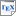[JKNT09b] M. Jurdzinski, M. Kwiatkowska, G. Norman and A. Trivedi. Concavely-Priced Probabilistic Timed Automata. Technical report RR-09-06, Oxford University Computing Laboratory. 2009. [pdf] [bib] Downloads:pdf (325 KB)bib Notes: An full version of this paper with proofs can be found in [JKNT09] Links: [Google] [Google Scholar] [CiteSeer] Abstract. Concavely-priced probabilistic timed automata, an extension of probabilistic timed automata, are introduced. In this paper we consider expected reachability, discounted, and average price problems for concavely-priced probabilistic timed automata for arbitrary initial states. We prove that these problems are EXPTIME-complete for probabilistic timed automata with two or more clocks and PTIME-complete for automata with one clock. Previous work on expected price problems for probabilistic timed automata was restricted to expected reachability for linearly-priced automata and integer valued initial states. This work uses the boundary region graph introduced by Jurdzinski and Trivedi to analyse properties of concavely-priced (non-probabilistic) timed automata.